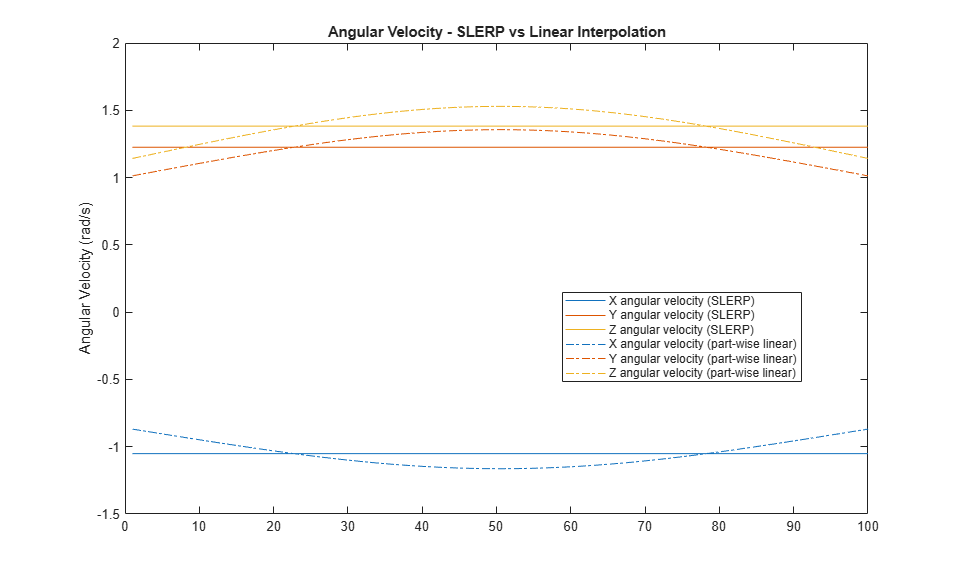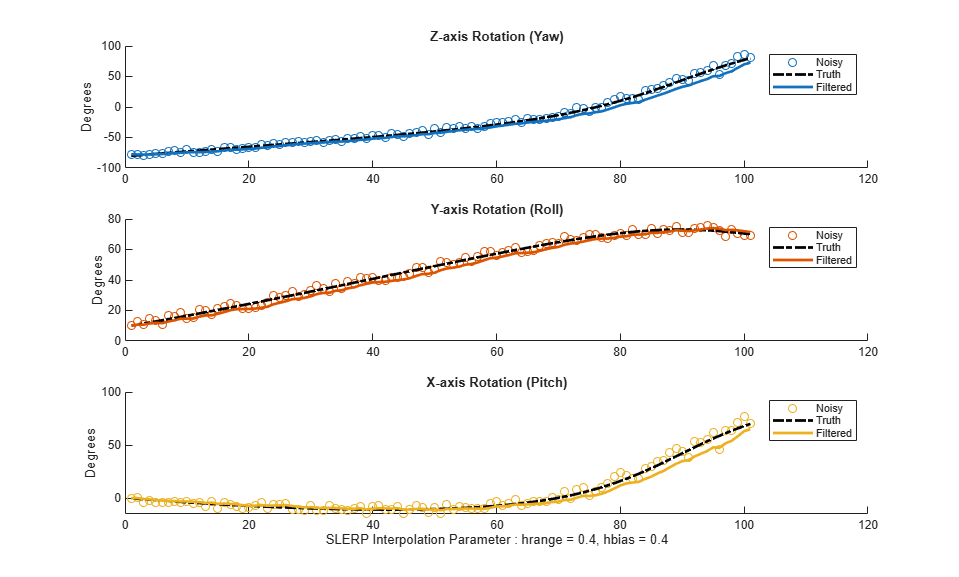Documentation

## Lowpass Filter Orientation Using Quaternion SLERP

This example shows how to use spherical linear interpolation (SLERP) to create sequences of quaternions and lowpass filter noisy trajectories. SLERP is a commonly used computer graphics technique for creating animations of a rotating object.

### SLERP Overview

Consider a pair of quaternionsand. Spherical linear interpolation allows you to create a sequence of quaternions that vary smoothly betweenandwith a constant angular velocity. SLERP uses an interpolation parameter `h` that can vary between 0 and 1 and determines how close the output quaternion is to eitheror.

The original formulation of quaternion SLERP was given by Ken Shoemake [ 1] as:

``

An alternate formulation with sinusoids (used in the `slerp` function implementation) is:

``

whereis the dot product of the quaternion parts. Note that.

### SLERP vs Linear Interpolation of Quaternion Parts

Consider the following example. Build two quaternions from Euler angles.

```q0 = quaternion([-80 10 0], 'eulerd', 'ZYX', 'frame'); q1 = quaternion([80 70 70], 'eulerd', 'ZYX', 'frame'); ```

To find a quaternion 30 percent of the way from `q0` to `q1`, specify the `slerp` parameter as 0.3.

```p30 = slerp(q0, q1, 0.3); ```

To view the interpolated quaternion's Euler angle representation, use the `eulerd` function.

```eulerd(p30, 'ZYX', 'frame') ```
```ans = -56.6792 33.2464 -9.6740 ```

To create a smooth trajectory between `q0` and `q1`, specify the `slerp` interpolation parameter as a vector of evenly spaced numbers between 0 and 1.

```dt = 0.01; h = (0:dt:1).'; trajSlerped = slerp(q0, q1, h); ```

Compare the results of the SLERP algorithm with a trajectory between `q0` and `q1`, using simple linear interpolation (LERP) of each quaternion part.

```partsLinInterp = interp1( [0;1], compact([q0;q1]), h, 'linear'); ```

Note that linear interpolation does not give unit quaternions, so they must be normalized.

```trajLerped = normalize(quaternion(partsLinInterp)); ```

Compute the angular velocities from each approach.

```avSlerp = helperQuat2AV(trajSlerped, dt); avLerp = helperQuat2AV(trajLerped, dt); ```

Plot both sets of angular velocities. Notice that the angular velocity for SLERP is constant, but it varies for linear interpolation.

```sp = HelperSlerpPlotting; sp.plotAngularVelocities(avSlerp, avLerp); ```SLERP produces a smooth rotation at a constant rate.

### Lowpass Filtering with SLERP

SLERP can also be used to make more complex functions. Here, SLERP is used to lowpass filter a noisy trajectory.

Rotational noise can be constructed by forming a quaternion from a noisy rotation vector.

```rcurr = rng(1); sigma = 1e-1; noiserv = sigma .* ( rand(numel(h), 3) - 0.5); qnoise = quaternion(noiserv, 'rotvec'); rng(rcurr); ```

To corrupt the trajectory `trajSlerped` with noise, incrementally rotate the trajectory with the noise vector `qnoise`.

```trajNoisy = trajSlerped .* qnoise; ```

You can smooth real-valued signals using a single pole filter of the form:

``

This formula essentially says that the new filter stateshould be moved toward the current inputby a step size that is proportional to the distance between the current input and the current filter state.

The spirit of this approach informs how a quaternion sequence can be lowpass filtered. To do this, both the `dist` and `slerp` functions are used.

The `dist` function returns a measurement in radians of the difference in rotation applied by two quaternions. The range of the `dist` function is the half-open interval [0, pi).

The `slerp` function is used to steer the filter state towards the current input. It is steered more towards the input when the difference between the input and current filter state has a large `dist`, and less toward the input when `dist` gives a small value. The interpolation parameter to `slerp` is in the closed-interval [0,1], so the output of `dist` must be re-normalized to this range. However, the full range of [0,1] for the interpolation parameter gives poor performance, so it is limited to a smaller range `hrange` centered at `hbias`.

```hrange = 0.4; hbias = 0.4; ```

Limit `low` and `high` to the interval [0, 1].

```low = max(min(hbias - (hrange./2), 1), 0); high = max(min(hbias + (hrange./2), 1), 0); hrangeLimited = high - low; ```

Initialize the filter and preallocate outputs.

```y = trajNoisy(1); % initial filter state qout = zeros(size(y), 'like', y); % preallocate filter output qout(1) = y; ```

Filter the noisy trajectory, sample-by-sample.

```for ii=2:numel(trajNoisy) x = trajNoisy(ii); d = dist(y, x); % Renormalize dist output to the range [low, high] hlpf = (d./pi).*hrangeLimited + low; y = slerp(y,x,hlpf); qout(ii) = y; end f = figure; sp.plotEulerd(f, trajNoisy, 'o'); sp.plotEulerd(f, trajSlerped, 'k-.', 'LineWidth', 2); sp.plotEulerd(f, qout, '-', 'LineWidth', 2); sp.addAnnotations(f, hrange, hbias); ```### Conclusion

SLERP can be used for creating both short trajectories between two orientations and for smoothing or lowpass filtering. It has found widespread use in a variety of industries.

### References

1. Shoemake, Ken. "Animating Rotation with Quaternion Curves." ACM SIGRAPH Computer Graphics 19, no 3 (1985):245-54, doi:10.1145/325165.325242

##### SupportGet trial now